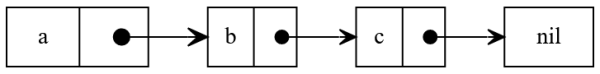## Data structures, simple single linked lists using Go

We already talked about queues and how to implement them using arrays and “lists” so I think I should expand a little more about why we do the differentiation between arrays (or vectors) and lists.

A big difference between linked lists (not list, I will clarify later why) and vectors or arrays is the memory usage. In an array (or vector) we allocate the whole size of the array in the moment we create the array, for example, if we create an array of integers with 10 members we will immediately allocate a continous block of memory of a size of 10 integers. This makes arrays difficult to scale if we are adding or removing elements constantly, if we decide we will have a max of 100 elements but most of the time we contain only 15 we are wasting 85 element size spaces in nothing but if we decide to store more than 100 elements then we will have to resize the array and that will require request another chunk of memory and then relocate all the elements to their new addresses, not a really nice thing to be taken care of.

A work around for this is using a linked list, in its simplest form it is just a node that points to the next node, if you read my blog post about implementing lists in OCaml from scratch it is simple to do in a functional language like OCaml:

type 'a seq =
| End
| Next of 'a * a' seq
;;


There are many different types of linked lists (in fact, I usually find more different types in literature every week) but the most common is the single linked list where a node only points to the next node in the list or to nothing if it is the last one in the list (there are many variations of this mix, I will probably mention them in a separate post in the future).Because implementing a linked list in a functional programming is kind of cheating for me (you saw the implementation in OCaml) I will do in a more close to real language, Go:

type node struct {
data string
next *node
}

top *node
}

func (list *LinkedList) IsEmpty() bool {
return list.top == nil
}

func (list *LinkedList) Append(element string) {
n := node{data: element}
if list.top == nil {
list.top = &n
return
}

current := list.top
for current.next != nil {
current = current.next
}
current.next = &n
}

func (list *LinkedList) Prepend(element string) {
n := node{data: element}
if list.top == nil {
list.top = &n
return
}

n.next = list.top
list.top = &n
}


As you see, appending (adding an element at the end) with our simple list is a very expensive operation, $$\Theta(n)$$, while prepending (adding an element at the beginning) is always $$\Theta(1)$$. Yes, there are ways we can amortize this, for example using a pointer for the tail of the list but for now I will keep it simple to illustrate my point, in fact, to do the most basic linked list we don’t even need a separate structure (as here with LinkedList) because only knowing the first node is enough to go around the whole list, that way is actually really common instead of using a whole completely alternate structure, a linked list is more an implementation (data structure) than an abstract data type.

I made the previous distinction because a common question I get all the time is “when should we implement our own data structure from scratch?” and to be honest my answer, 99% of the time, would be never, just use the data structures including in your standard libraries but there is usually that 1% that needs you to know “oh, this actually looks like a problem where I just need to point to the first element and then that will point to the next and so on because I will always only add elements at the beginning” so in your mind you know you should implement a data structure named a linked list, see? it is not in vain learning all these banal stuff, believe me, one day it will save the day ;)

As usual I leave you here with the test implementation, I just used here the testify external library because honestly, I dislike how verbose unit tests are in Go. I leave to you to implement the remove and search operations (spoiler alert, it will be a $$\mathcal{O}(n)$$ operation).

import (
"testing"
"github.com/stretchr/testify/assert"
)

func TestEmptyList(t *testing.T) {

assert.True(t, list.IsEmpty())
assert.Nil(t, list.top)
}

func TestEmptyListAppendOneItem(t *testing.T) {
list.Append("a")

assert.NotNil(t, list.top)
assert.Equal(t, list.top.data, "a")
assert.Nil(t, list.top.next)
assert.False(t, list.IsEmpty())
}

func TestListAppendsAtTheEnd(t *testing.T) {
list.Append("a")
list.Append("b")

assert.NotNil(t, list.top.next)
assert.Nil(t, list.top.next.next)
assert.Equal(t, list.top.data, "a")
assert.Equal(t, list.top.next.data, "b")
}

func TestListPrependsAtTheBeginning(t *testing.T) {